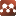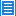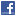## Mathematical Physics## A Topological Transformation of Quantum Dynamics

Authors: Vu B Ho

In this work we discuss the topological transformation of quantum dynamics by showing the wave dynamics of a quantum particle on different types of topological structures in various dimensions from the fundamental polygons of the corresponding universal covering spaces. This is not the view from different perspectives of an observer who simply uses different coordinate systems to describe the same physical phenomenon but rather possible geometric and topological structures that quantum particles are endowed with when they are identified with differentiable manifolds that are embedded or immersed in Euclidean spaces of higher dimension. We present our discussions in the form of Bohr model in one, two and three dimensions using linear wave equations. In one dimension, the fundamental polygon is an interval and the universal covering space is the straight line and in this case the standing wave on a finite string is transformed into the standing wave on a circle which can be applied into the Bohr model of the hydrogen atom. In two dimensions, the fundamental polygon is a square and the universal covering space is the plane and in this case the standing wave on the square is transformed into the standing wave on different surfaces that can be formed by gluing opposite sides of the square, which include a 2-sphere, a 2-torus, a Klein bottle and a projective plane. In three dimensions, the fundamental polygon is a cube and the universal covering space is the three-dimensional Euclidean space. It is shown that a 3-torus and the manifold K×S^1 defined as the product of a Klein bottle and a circle can be constructed by gluing opposite faces of a cube therefore in three-dimensions the standing wave on a cube is transformed into the standing wave on a 3-torus or on the manifold K×S^1. We also suggest that the mathematical degeneracy may play an important role in quantum dynamics and be associated with the concept of wavefunction collapse in quantum mechanics.

Comments: 26 Pages. This paper has been published in Journal of Modern Physics

### Submission history

[v1] 2018-10-20 04:55:25
[v2] 2019-02-14 01:38:54